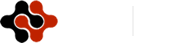International Journal of Mathematics and Computational Science, Vol. 1, No. 2, April 2015 Publish Date: Mar. 31, 2015 Pages: 55-58

New Method of the Approximation of Hartree-Fock Wave Functions

N. V. Novikov*

Skobeltsyn Institute of Nuclear Physics, Moscow State University, Moscow, Russia

Abstract

A new method of the approximation of the solution to the Hartree-Fock equation for many-electron atoms and ions is proposed. The method is based on the increase in the accuracy of the approximation of wave functions corresponding to different shells. This makes it possible to reach the accuracy that is close to the error of the numerical solution. More than 14000 wave functions of the ground and excited states of atoms and ions with the number of electrons ranging from 2 to 18 are obtained.

Keywords

Hartree-Fock Equation, Wave Function, Excited States of Ions

Contents

1. Introduction

Hartree-Fock atomic wave functions are independent-particle-model approximations to the nonrelativistic Schrodinger equation for stationary states [1,2]. The Pauli principle is imposed through the use of Slater determinants, and for an N-electron system, the Hartree--Fock equations yield N Hartree--Fock spin orbitals.

In the conventional Hartree--Fock approximation, the spin orbitals are expressed as products of a radial function times a spherical harmonic times a spin function.  Radial functions are taken to depend only on the quantum numbers  n and l, and the total wave function is required to be an eigenfunction of the total orbital and spin angular  momentum; the form of the spin orbitals guarantees that L, S and parity are good quantum numbers.

Hartree--Fock wave functions for atoms may be computed numerically by standard methods . Multi Configuration Hartree Fock (MCHF) program  allows obtaining the numerical solution of the nonrelativistic Hartree--Fock equation for ground and excited states of atoms and ions. MCHF program is very efficient for treating correlation in a large variety of systems. MCHF calculation of total energy takes into account the hyperfine structure and relativistic effects. Progress in atomic transition probability calculations has been made offering the possibility of separate and independent optimization of the initial and final configuration spaces. The disadvantage of numerical wave functions is that nontrivial software is required for their using.

Roothaan-Hartree-Fock wave functions [5,6] are approximations to conventional Hartree-Fock wave functions in which the radial atomic orbitals are expanded as a finite superposition of primitive radial functions. These wave functions offer some advantages over their numerical counterparts as they can be readily incorporated into a variety of codes for atomic calculations, and also for molecular and solid-state calculations by density-functional methods. The Roothaan-Hartree-Fock atomic wave functions are useful as starting points for relativistic calculations and in atomic structure calculations for atomic physics and quantum chemistry.

The method for analytically approximating the numerical solution of the Hartree--Fock equation by means of the combination of Slater orbitals was used for the wave functions of the ground states of negative ions with the nuclear charges Z≤16  and atoms with the nuclear charges Z≤54  and Z=55  and 55≤Z≤92  and Z≤54  and ions with Z≤18 . The paper  is cited more than 3000 times. However, using this method for approximating the wave functions of the excited states of atoms and ions leads to a significant increase in the estimation error.

The aim of this paper is to propose a new method for obtaining the wave functions in the Hartree--Fock approximation, which makes it possible to represent the wave functions of the excited states of atoms and ions in the analytical form and carry out the calculation for the lower excited states of atoms and ions with the nuclear charges Z≤18.

We let the function φnl(r), where n is the principal quantum number of the electron, l is its orbital number, and r is the radial variable, denote the numerical solution of the radial Hartree--Fock equation.  The function φnl(r) must satisfy the orthogonality conditions:

< φnl(r) │ φnl(r) > = δnn.                          (1)

Because of the error of the numerical solution to the Hartree-Fock equation, this equality holds only approximately:

< φnl(r) │ φnl(r) > = hn,                         (2)

where │hn’│ << 1 for n’< n and hn’ = 1 for n’= n.

The problem of the approximation of the function φnl(r) consists in finding the function ψnl(r) that is expressed in terms of the analytical functions with the least error Δnl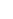Δnl = ∫dr r2 │ φnl(r) - ψnl(r) │2                (3)

and satisfies the orthogonality conditions as well as possible:

< ψnl(r) │ ψn’l(r) > = xn’,                   (4)

where  │xn’│ << 1   for n’< n and xn’ = 1 for  n’= n

To do this, it is possible to use the expansion ψnl(r) in terms of the basis of Slater orbitals:

ψnl(r) =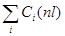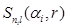,                (5)

where Snl(α,r) =  rn-l  exp(-α r) and Ci(nl) are the expansion coefficients.

In what follows, as an example, we consider the approximation of the numerical solution to the Hartree--Fock equation for the M1 shell of the atom or ion that has 10 electrons or more.  We first find the parameters of the function corresponding minimum energy for the given l:

ψ1s(r) =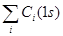,                 (6)

where the parameters Ci(1s),  ni,  and αi  are calculated from the minimum of functional (3) with the functions φ1s(r) and ψ1s(r). Then, as a test function for the approximation of φ2s(r), we chose

ψ2s(r) =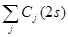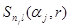-

<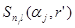ψ1s(r’) > ψ1s(r) ,           (7)

which, by construction, satisfies the orthogonality condition

< ψ2s(r) │ψ1s(r) > =0.                           (8)

The parameters Cj(2s), nj, and αj in (7) are determined from the minimum of functional (3) with the functions φ2s(r) and ψ2s(r).  Now, as a test function for the approximation of φ3s(r), we chose the function

ψ3s(r) =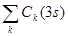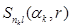-

<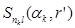│ψ1s(r’) > ψ1s(r)

- <│ψ2s(r) > ψ2s(r),       (9)

which, by construction, satisfies the orthogonality conditions:

< ψ3s(r) │ψ1s(r) > =0,

<  ψ3s(r) │ψ2s(r) > =0.                         (10)

The parameters Ck(3s),  nk,  and αk  in (9) are determined from the minimum  of functional (3) with the functions φ3s(r) and ψ3s(r).

The advantage of this approximation method consists in that the set of orthonormalized functions is obtained. However, the rounding of the coefficients Сj in (7) and Сk in (9) leads to the fulfillment of (10) only with an error xn’¹0 (4). The drawback of this method is that the approximation error Δnl increases rapidly with increasing n. The increase in the number of shells in the atom or ion leads to an increase in the number of additional conditions imposed on the test function.

A strong correlation between the parameters Сi(nl) and αi exists in expansions (6), (7), (9). The variation in the value of one of the parameters in these expansions leads to a change in all Сi(nl) and  αi,  which makes it difficult to find the minimum of the multiparameter function. In addition, the overlap integrals in (7) and (9), which also depend on the varied parameters, become a strong perturbation. Such a "feedback" also makes it difficult significantly to perform the variation of functional (3) and leads to an increase in the error Δnl. The variation of the parameters Ck(3s) and  αk in (9) must not only decrease the deviation of the first term in (9) from  φ3s(r), but also decrease overlap integrals (10) in order that they remains corrections to the first term in (9).

This approximation method was used previously [7-11] to describe the ground state of atoms and ions and was not applied to excited states of many-electron atoms and ions.

3. New Approximation Method

We propose to refuse to choose the test function with conditions (1) taken into account and thereby to decrease Δnl. If the errors Δnl are rather small, then orthogonality conditions (4) are satisfied with the error that is close to that of numerical solution (2):

xn│ → │hn│.                                (11)

For the case of the approximation of the wave function of the М1 shell, the test function for the K shell has the same form (6), and for the states with higher  energies in absolute value, the test functions were chosen in the forms

ψ2s(r) =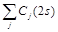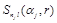;

ψ3s(r) =.             (12)

Because any additional conditions are imposed on functions (12), then it is possible to obtain small Δ2s and Δ3s such that conditions are satisfied (11).

Based on the proposed method, we calculated the wave functions of atoms and ions with the nuclear charge Z≤Zmax, where Zmax=18, and the number of electrons Ne (2 £ Ne £ 18). In addition to the ground states, we also took into account the states with one excited valence electron in the case where there are no vacancies in the inner shells. We considered the following excited states: 2s for Z=2, 2p for Z4, 3s for Z≤10, and 3p for Z≤12, as well as the 3d, 4s, 4p, 4d, 5s, 5p, and 5d states for Z≤18. For atoms and ions with one valence electron (Ne = 3, 11, 13), the spin in the excited state remains the same as in the ground state. Two possible values of the spin for the excited states are taken into account in the other cases.

The approximation accuracy Δnl in the calculations was as follows: Δ1s≤ 3´10-10, Δnl≤ 10-9 for n=2 and Δnl≤ 3´10-9 for  n≥3. This accuracy Δnl is sufficient for condition (11) to be satisfied. In this case, the obtained value of │xn’│for atoms and ions in the ground state turns out to be less than that for the wave functions [8,11]. As a result, more than 14000 wave functions were obtained:

Nwf  =  NZ ( Nex  Nnl + Nnl - 1) ,                  (13)

where Nwf are the number of wave functions,  NZ is the number of atoms and positive ions (NZ= Zmax-Ne+1), Nnl is the maximum number of shells, and Nex is the number of considered excited states.  The value of Nnl ,  Nex, and Nwf    are given in the table.

The results of calculations (the parameters of wave functions) are represented in free access . These wave functions are useful for atomic calculations [13,14].

4. Conclusions

We have proposed a new method for approximating the solution to the Hartree--Fock equation for many-electron atoms and ions. The method is based on the increase in the approximation accuracy for the wave functions of electrons of all shells. This makes it possible to obtain the accuracy for the wave functions that is close to that of the numerical solution. We were first to obtain more than 14000 wave functions of the excited states of atoms and ions with nuclear charge Z≤18 and with  the number of electrons ranging from 2 to 18 in the form of the expansion in terms of analytical functions. For atoms and ions with one valence electron (Ne = 3, 11, 13), the spin in the excited state remains the same as in the ground state. Two possible values of the spin for the excited states are taken into account in the other cases.

Acknowledgments

The author is grateful to Ya. A. Teplova for the useful remarks during the discussion of the results of this paper.

Dependence of the number of atoms and positive ionsNZ, the values of the parameters Nnl  and Nex, and the number of obtained wave functions Nwf  on the number of electrons Ne

 Ne NZ Nnl Nex Nwf 2 17 2 22 765 3 16 2 10 336 4 15 3 21 975 5 14 3 9 406 6 13 4 42 2223 7 12 4 42 2052 8 11 4 20 913 9 10 4 42 1710 10 9 4 42 1539 11 8 4 8 280 12 7 5 15 553 13 6 5 7 234 14 5 6 30 925 15 4 6 30 740 16 3 6 14 267 17 2 6 30 370 18 1 6 30 185

References

1. D.R. Hartree, The Calculations of Atomic Structures, Wiley, New York, 1957.
2. J.S. Slater, Quantum Theory of Atomic Structures, Vol.1 and 2, McGraw-Hill, New York 1960.
3. C. Froese Fischer, The Hartree-Fock Method for atoms, Wiley, New York, 1977.
4. C. Froese Fischer, Computer Phys. Commun. 64 (1991) 369-519.
5. C. C. Roothan, Rev. Mod. Phys. 23 (1951) 69-89
6. C. C. Roothan, Rev. Mod. Phys. 32 (1960) 179-192.
7. S. Huzinaga, A. Hart-Davis, Phys. Rev. A 8 (1973) 1734-1738.
8. E. Clementi, C. Roetti, Atomic Data Nucl.Data Tables.  14 (1974)  177-478.
9. M.L. Sachdeva, S.P. Puri, Phys.Rev. A 14 (1976) 1603-1606.
10. A.D. McLean, R.S. McLean,Atomic Data Nucl. Data Tables.  26 (1981)  197-381.
11. C.F. Bunge, J.A. Barrientos, A.V. Bunge.  Atomic Data Nucl. Data Tables 53 (1993) 113-162.
12. N.V. Novikov, Wave Function Value Database http://cdfe.sinp.msu.ru/services/wftables
13. N.V. Novikov, Ya. A. Teplova, Physics Letters A 375 (2011) 4138-4140.
14. N.V. Novikov,Journal of Surface Investigation. X_ray, Synchrotron and Neutron Techniques, 6 (2012) 203–207.

 Contents 1. 2. 3. 4.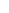600 ATLANTIC AVE, BOSTON,
MA 02210, USA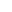+001-6179630233
JournalsJournal ListsAuthor GuidelinesPayment InformationSubmit a ManuscriptOpen Special IssuesJoin as Editorial MembersJoin as ReviewersPrivacy NoticeTerms of ConditionsHelp Center